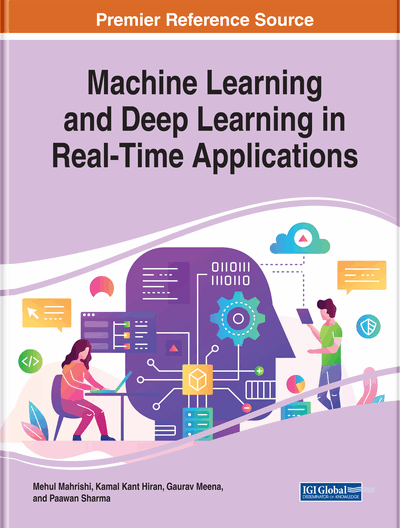# Text Separation From Document Images: A Deep Learning Approach

Priti P. Rege (College of Engineering, Pune, India) and Shaheera Akhter (Government College of Engineering, Pune, India)
DOI: 10.4018/978-1-7998-3095-5.ch013

## Abstract

Text separation in document image analysis is an important preprocessing step before executing an optical character recognition (OCR) task. It is necessary to improve the accuracy of an OCR system. Traditionally, for separating text from a document, different feature extraction processes have been used that require handcrafting of the features. However, deep learning-based methods are excellent feature extractors that learn features from the training data automatically. Deep learning gives state-of-the-art results on various computer vision, image classification, segmentation, image captioning, object detection, and recognition tasks. This chapter compares various traditional as well as deep-learning techniques and uses a semantic segmentation method for separating text from Devanagari document images using U-Net and ResU-Net models. These models are further fine-tuned for transfer learning to get more precise results. The final results show that deep learning methods give more accurate results compared with conventional methods of image processing for Devanagari text extraction.
Chapter Preview
Top

## Background

Document text segmentation uses three approaches: top-down, bottom-up, and a hybrid approach. Each of the three approaches, top-down, bottom-up, and hybrid, in turn, consists of three steps: pre-processing, segmentation, and classification or feature extraction. The deep learning approach does not require pre-processing and feature extraction steps. Some work has been carried out using the deep learning approach by making use of Semantic Segmentation. Literature reviewed explains text segmentation using top-down, bottom-up, hybrid, and deep learning approaches.

## Key Terms in this Chapter

True Positive (TP): It is the number of abnormal samples detected as abnormal samples by the classifier algorithm.

False Positive (FP): It is the number of normal samples detected as abnormal samples by the classifier algorithm.

True Negative (TN): It is the number of normal samples detected as normal samples by the classifier algorithm.

Specificity: Specificity is a percentage of correctly classified normal samples, and it is given by the ratio of true negative to the addition of true negative and false positive samples.

Precision: Precision is the ratio of correctly predicted positive observations of the total predicted positive observations. Precision = TP/TP+FP.

F1 Score: F1 score is the weighted average of precision and recall. Therefore, this score takes both false positives and false negatives into account. Intuitively it is not as easy to understand as accuracy, but F1 is usually more useful than accuracy, especially if you have an uneven class distribution. Accuracy works best if false positives and false negatives have similar cost. If the value of false positives and false negatives are very different, it's better to look at both Precision and Recall. F1 Score = 2*(Recall * Precision) / (Recall + Precision).

Accuracy: Accuracy is a percentage of correctly classified normal as well as abnormal samples out of total samples. It is given by the ratio of the addition of True Positive and True Negative samples to the total number of samples under test. Accuracy = TP+TN/TP+FP+FN+TN.

Recall (Sensitivity): Recall is the ratio of correctly predicted positive observations to all observations in actual class - yes. Recall = TP/TP+FN.

Sensitivity: Sensitivity is a percentage of correctly classified abnormal samples, and it is given by the ratio of true positive to the addition of true positive and false negative samples.

False Negative (FN): It is the number of abnormal samples detected as normal samples by the classifier algorithm.

## Complete Chapter List

Search this Book:
Reset